Reproducing BMA

2020-11-10

By default, the package estimates an ensemble of 12 meta-analytic models and provides functions for convenient manipulation with the fitted object. However, it has been built in a way that it can be used as a framework for estimating any combination of meta-analytic models (or a single model). Here, we illustrate how to build a custom ensemble of meta-analytic models - specifically the same ensemble as is used in ‘classical’ Bayesian Meta-Analysis (Gronau et al., 2017). See this vignette if you are interested in building more customized ensembles.

Reproducing Bayesian Meta-Analysis (BMA)

We illustrate how to fit a classical BMA (not adjusting for publication bias) using RoBMA. For this purpose, we reproduce a meta-analysis of registered reports on Power posing by Gronau et al. (2017). We focus only on the analysis using all reported results using a Cauchy prior distribution with scale $$1/\sqrt{2}$$ for the effect size estimation (half-Cauchy for testing) and inverse-gamma distribution with scale = 1 and shape 0.15 for the heterogeneity parameter. You can find the figure from the original publication here and the paper’s supplementary materials at https://osf.io/fxg32/.

First, we load the power posing data provided within the metaBMA package and reproduce the analysis performed by Gronau et al. (2017).

data("power_pose", package = "metaBMA")
power_pose[,c("study", "effectSize", "SE")]
#>                study effectSize        SE
#> 1      Bailey et al.  0.2507640 0.2071399
#> 2       Ronay et al.  0.2275180 0.1931046
#> 3 Klaschinski et al.  0.3186069 0.1423228
#> 4     Bombari et al.  0.2832082 0.1421356
#> 5        Latu et al.  0.1463949 0.1416107
#> 6      Keller et al.  0.1509773 0.1221166
fit_BMA_test <- metaBMA::meta_bma(y   = power_pose$effectSize, SE = power_pose$SE,
d   = metaBMA::prior(family = "halfcauchy", param = 1/sqrt(2)),
tau = metaBMA::prior(family = "invgamma", param = c(1, .15)))

fit_BMA_est  <- metaBMA::meta_bma(y   = power_pose$effectSize, SE = power_pose$SE,
d   = metaBMA::prior(family = "cauchy", param = 1/sqrt(2)),
tau = metaBMA::prior(family = "invgamma", param = c(1, .15)))
fit_BMA_test$inclusion #> ### Inclusion Bayes factor ### #> Model Prior Posterior included #> 1 fixed_H0 0.25 0.00868 #> 2 fixed_H1 0.25 0.77745 x #> 3 random_H0 0.25 0.02061 #> 4 random_H1 0.25 0.19325 x #> #> Inclusion posterior probability: 0.971 #> Inclusion Bayes factor: 33.136 round(fit_BMA_est$estimates,2)
#>          mean   sd 2.5%  50% 97.5% hpd95_lower hpd95_upper  n_eff Rhat
#> averaged 0.22 0.06 0.09 0.22  0.34        0.09        0.34     NA   NA
#> fixed    0.22 0.06 0.10 0.22  0.34        0.10        0.34 3693.1    1
#> random   0.22 0.08 0.07 0.22  0.37        0.07        0.37 5041.1    1

From the output, we can see that the inclusion Bayes factor for the effect size was $$BF_{10} = 33.14$$ and the effect size estimate 0.22, 95% HDI [0.09, 0.34] which matches the reported results. Please note that the metaBMA package model-averages only across the $$H_{1}$$ models, whereas the RoBMA package model-averages across all models.

Using RoBMA

Now we reproduce the analysis with RoBMA. Note that some differences in the results are expected since the RoBMA package evaluates the likelihood of test-statistics and not the effect sizes itself. The original data contain t-values and sample sizes, which would be the preffered input, however, we use the general effect sizes and standard errors input to show that the RoBMA() can handle them as well. We set the corresponding prior distributions for effect sizes ($$\mu$$) and heterogeneity ($$\tau$$), and remove the alternative prior distributions for the publication bias ($$\omega$$) by setting priors_omega = NULL. Note that for specifying half-Cauchy prior distribution with the RoBMA::prior() function, we use a regular Cauchy distribution and truncate it at zero. The inverse-gamma prior distribution for the $$\tau$$ parameter is the default option (we specify it for completeness) and we omit the specifications for the null prior distributions for $$\mu$$, $$\tau$$ (both of which are set to a spike at 0 by default), and $$\omega$$ (which is set to no publication bias by default). We also turn on the silent option to not spam the output and set a seed for reproducibility.

library(RoBMA)

fit_RoBMA_test <- RoBMA(y = power_pose$effectSize, se = power_pose$SE, study_names = power_pose$study, priors_mu = prior( distribution = "cauchy", parameters = list(location = 0, scale = 1/sqrt(2)), truncation = list(0, Inf)), priors_tau = prior( distribution = "invgamma", parameters = list(shape = 1, scale = 0.15)), priors_omega = NULL, control = list(silent = TRUE), seed = 1) fit_RoBMA_est <- RoBMA(y = power_pose$effectSize, se = power_pose$SE, study_names = power_pose$study,
priors_mu  = prior(
distribution = "cauchy",
parameters = list(location = 0, scale = 1/sqrt(2))),
priors_tau = prior(
distribution = "invgamma",
parameters = list(shape = 1, scale = 0.15)),
priors_omega = NULL,
control = list(silent = TRUE), seed = 2)
summary(fit_RoBMA_test)
#> Call:
#> RoBMA(y = power_pose$effectSize, se = power_pose$SE, study_names = power_pose$study, #> priors_mu = prior(distribution = "cauchy", parameters = list(location = 0, #> scale = 1/sqrt(2)), truncation = list(0, Inf)), priors_tau = prior(distribution = "invgamma", #> parameters = list(shape = 1, scale = 0.15)), priors_omega = NULL, #> control = list(silent = TRUE), seed = 1) #> #> Robust Bayesian Meta-Analysis #> Models Prior prob. Post. prob. Incl. BF #> Effect 2/4 0.500 0.971 33.101 #> Heterogeneity 2/4 0.500 0.214 0.272 #> Pub. bias 0/4 0.000 0.000 NA #> #> Model-averaged estimates #> Mean Median 0.025 0.975 #> mu 0.213 0.217 0.000 0.345 #> tau 0.021 0.000 0.000 0.169 summary(fit_RoBMA_est, conditional = TRUE) #> Call: #> RoBMA(y = power_pose$effectSize, se = power_pose$SE, study_names = power_pose$study,
#>     priors_mu = prior(distribution = "cauchy", parameters = list(location = 0,
#>         scale = 1/sqrt(2))), priors_tau = prior(distribution = "invgamma",
#>         parameters = list(shape = 1, scale = 0.15)), priors_omega = NULL,
#>     control = list(silent = TRUE), seed = 2)
#>
#> Robust Bayesian Meta-Analysis
#>               Models Prior prob. Post. prob. Incl. BF
#> Effect           2/4       0.500       0.943   16.603
#> Heterogeneity    2/4       0.500       0.228    0.295
#> Pub. bias        0/4       0.000       0.000       NA
#>
#> Model-averaged estimates
#>      Mean Median 0.025 0.975
#> mu  0.209  0.216 0.000 0.349
#> tau 0.025  0.000 0.000 0.190
#>
#> Conditional estimates
#>      Mean Median 0.025 0.975
#> mu  0.221  0.221 0.099 0.349
#> tau 0.109  0.090 0.031 0.302

At first, we notice a few warning messages which inform us about using data-informed starting values. These are harmless and are further discussed in another vignette

The output from the summary.RoBMA() function has 2 parts. The first one under the “Robust Bayesian Meta-Analysis” heading provides a basic summary of the fitted models by the component types (presence of Effect/Heterogeneity/Publication bias). Here, we can see that there are no models correcting for publication bias (we disabled them by setting priors_omega = NULL). We can find there the prior and posterior component probability and their inclusion BF. The results for the half-Cauchy model specified for testing show that the inclusion BF is basically identical to the one computed by the metaBMA package, $$BF_{10} = 33.10$$. The second part under the ‘Model-averaged estimates’ heading displays the parameter estimates (that are model-averaged across all fitted models. If we want to compare the results to the output from metaBMA, we have to look into the table under the ‘Conditional estimates’ heading. It presents estimates averaged only across the models that assume the presence of the specific component (we invoke this section by adding conditional = TRUE argument). Here we can see, the conditional effect size estimate 0.22, 95% CI [0.10, 0.35] that mirrors the estimate from the metaBMA package.

Visualizating the results

RoBMA provides extensive options for visualizing the results. Here, we visualize the prior (grey) and posterior (black) distribution for the mean parameter. Note that this figure displays the model-averaged results by default, which contain weighted estimates from all of the models. The arrows stand for the probability of a spike, here, at the value 0. We can see on the left-side y-axis that the probability of the value 0 decreased from .50, to 0.06 (also obtainable from the “Robust Bayesian Meta-Analysis” field in the summary.RoBMA() function).

plot(fit_RoBMA_est, parameter = "mu", prior = TRUE)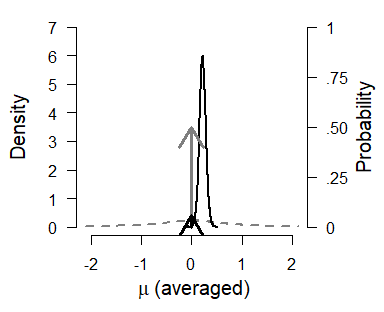Or, we can focus only on the conditional estimates, assuming that the prior distributions specified under the alternative hypothesis for $$\mu$$ are true by adding type = "conditional" argument. All of the figures can be also generated using the ggplot2 package, that allows further styling. To do that, we just need to add plot_type = "ggplot" to the plotting function call.

plot(fit_RoBMA_est, parameter = "mu", prior = TRUE, type = "conditional", plot_type = "ggplot")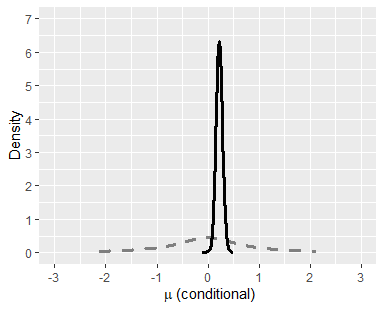We can also visualize the estimates from the individual models used in the ensemble. To do that, we need to change the type argument to type = "models". Whereas the first two models assume that the $$\mu$$ estimate is zero - we see their mean estimate as a square at zero, the last two models use the Cauchy distribution for prior on the mean parameter - we see their mean estimates and credible intervals. The size of the square denoting the mean estimate corresponds to its posterior probability, which is also displayed in the right-hand side panel. The bottom then shows the model averaged-estimate that weighted posterior distribution of all included models.

plot(fit_RoBMA_est, parameter = "mu", type = "models")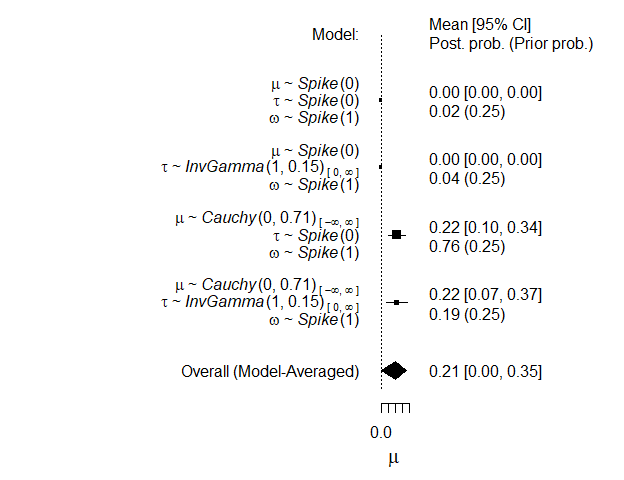The last type of visualization that we show here is the forest plot. It displays the original studies’ effects and the meta-analytic estimate within one figure. It can be requested by changing the parameter argument parameter = "forest".

plot(fit_RoBMA_est, parameter = "forest")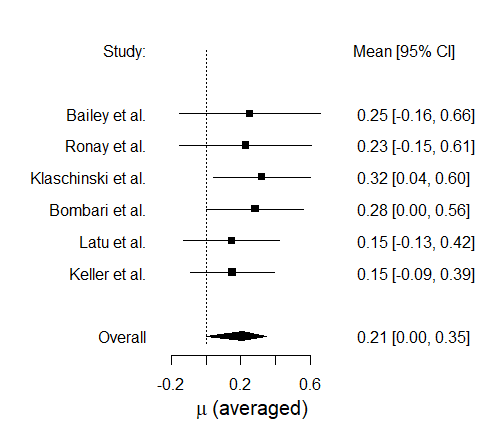Furthermore, we can include the estimated true effects of the studies in the figure as well by setting the parameter argument to parameter = c("forest","theta"). We can see that the true effect estimates (grey) are shrunk towards the meta-analytic estimate which is a result of the pooling occurring in the random effects models.

plot(fit_RoBMA_est, parameter = c("forest","theta"))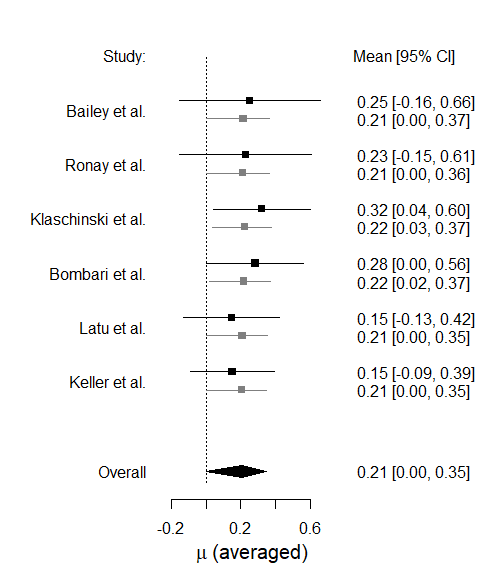For more options provided by the plotting function, see its documentation using plot.RoBMA().